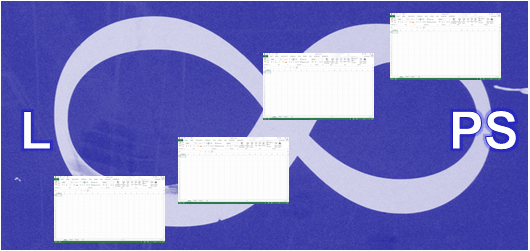Using loops to easily make changes across multiple worksheets

Loops are one of the key tools in Excel VBA when we need to perform tasks through a number of objects (cells, worksheets, charts, workbooks etc.) .  Here we will look at how to loop through Excel worksheets and workbooks.

Loop Through Excel WorksheetsBelow you will find three examples using different loops but all three will perform exactly the same task.

The For Each loop

1. An object variable (sh) is used and declared as Worksheet to tell Excel that we want store worksheets (the address) in the memory  of our computer (Dim sh As Worksheet).
2. The For Each loop will loop through each worksheet in the active workbook (For Each sh In ActiveWorkbook.Sheets).
3. The code will add 500 in A1 in all sheets in the active workbook.

Sub LoopSheets()
Dim sh As Worksheet
For Each sh In ActiveWorkbook.Sheets
sh.Range(“A1”).Value = 500
Next sh
End Sub

The For Next loop

1. A data variable is used to store a whole number (integer) in the computer’s memory (Dim iCounter As Integer).
2. The For Next loop is used to loop through all sheets in the active workbook but the loop needs to know how many worksheets there is in the active workbook (ActiveWorkbook.Sheets.Count).
3. The iCounter variable is used to move through the worksheets and the value 500 is entered in A1 in all worksheets in the active workbook (Sheets(iCounter).Range(“A1”).Value = 500).

Sub LoopSheetst2()
Dim iCounter As Integer
For iCounter = 1 To ActiveWorkbook.Sheets.Count
Sheets(iCounter).Range(“A1”).Value = 500
Next iCounter
End Sub

The Do loop

1. A data variable is used to store a whole number (integer) in the computer’s memory (Dim iCounter As Integer).
2. 1 is stored in the iCounter variable (iCounter = 1).
3. Do Until loop is used to run until criteria is met in this example until the value in the variable iCounter is total number of worksheets in the active workbook plus one (Do Until iCounter = ActiveWorkbook.Worksheets.Count + 1).

Sub LoopSheets3()
Dim iCounter As Integer
iCounter = 1
Do Until iCounter = ActiveWorkbook.Worksheets.Count + 1
Sheets(iCounter).Range(“A!”).Value = 500
iCounter = iCounter + 1
Loop
End Sub

Loop WorkbooksBelow you will find three examples using different loops but all three will perform exactly the same task but this time the loops will loop through workbooks.

The For Each loop

1. An object variable (wBook) is used and declared as Workbook to tell Excel that we want store workbooks (the address) in the memory of our computer (Dim WBook As Workbook).
2. The For Each loop will loop through each open workbook  (For Each wBook In Workbooks).
3. The code will add 2 in A2 in sheet 1 in all open workbooks.

Sub LoopWorkBooks()
Dim WBook As Workbook
For Each WBook In Workbooks
WBook.Sheets(1).Range(“A2”).Value = 2
Next WBook
End Sub

The For Next loop

1. A data variable is used to store a whole number (integer) in the computer’s memory (Dim iWB As Integer).
2. The For Next loop is used to loop through all open workbooks but the loop needs to know how many open workbooks we have (Workbooks.Count).
3. The iWB variable is used to move through the open workbooks and the value 2 is entered in A2 in sheet 1 in all open workbooks (Workbooks(iWB).Sheets(1).Range(“A2”).Value = 2).

Sub LoopWorkBooks2()
Dim iWB As Integer
For iWB = 1 To Workbooks.Count
Workbooks(iWB).Sheets(1).Range(“A2”).Value = 2
Next iWB
End Sub

The Do loop

1. A data variable is used to store a whole number (integer) in the computer’s memory (Dim iCounter As Integer).
2. 1 is stored in the iCounter variable (iCounter = 1).
3. Do Until loop is used to run until the criteria is met in this example until the value in the variable iCounter is total number of open workbooks plus one (Do Until iCounter = Workbooks.Count + 1).

Sub LoopWorkBooks3()
Dim iCounter As Integer
iCounter = 1
Do Until iCounter = Workbooks.Count + 1
Workbooks(iCounter).Sheets(1).Range(“A2”).Value = 2
iCounter = iCounter + 1
Loop
End Sub

Loop workbooks & worksheetsx

In the examples below nested loops are looping through workbooks and worksheets and again the For EachFor Next and the Do loop are used to do the job.

The For Each loop

Exactly as in the examples above in this post variables are used to store the address of the workbooks and worksheets in the computers memory (Dim WBook As Workbook & Dim sh As Worksheet). A For Each loop is used to run through the workbooks and one to run through the worksheets.

Sub LoopWorkBookSheets()
Dim WBook As Workbook
Dim sh As Worksheet
For Each WBook In Workbooks
For Each sh In WBook.Worksheets
sh.Range(“a1”) = 2
Next sh
Next WBook
End Sub

The For Next loop

Two For Next loops are needed to run through all worksheets in all open workbooks. Two variables are used (counter variables) to loop one workbook at the time and one worksheet.

Sub LoopWorkBookSheets2()
Dim iWB As Integer
Dim iCounter As Integer
For iWB = 1 To Workbooks.Count
For iCounter = 1 To Workbooks(iWB).Sheets.Count
Workbooks(iWB).Sheets(iCounter).Range(“b1”).Value = 450
Next iCounter
Next iWB
End Sub

The Do loop

It takes more coding to run through all worksheets in all open workbooks by using the Do loop. Again two loops are needed one for the workbooks and one for the worksheets.

Sub LoopWorkBookSheets3()
Dim iWorkBookCounter As Integer
Dim iSheetCounter As Integer
iWorkBookCounter = 1
iSheetCounter = 1
Do Until iWorkBookCounter = Workbooks.Count + 1
Do Until iSheetCounter = Workbooks(iWorkBookCounter).Sheets.Count + 1
Workbooks(iWorkBookCounter).Sheets(iSheetCounter).Range(“c1”).Value = 5
iSheetCounter = iSheetCounter + 1
Loop
iWorkBookCounter = iWorkBookCounter + 1
iSheetCounter = 1
Loop
End Sub

Some people prefer to use the For Each loop for a couple of reasons. The For Each loop is a faster loop and normally you need less coding.

Good luck with your loops!

Excel course is one of our Microsoft Office 365 training courses.

Useful Resources

Macro to Loop Through All Worksheets in a Workbook

Excel VBA 2013: Track Changes With the Inquire Add-In